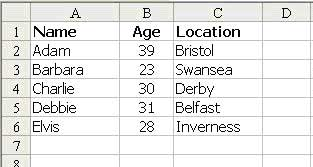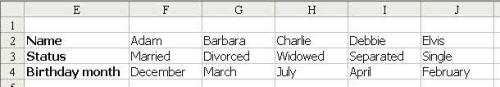# Excel Lookup Functions Explained

By

Ray BlakeUsing VLOOKUP, HLOOKUP, INDEX, and MATCH in Excel to interrogate data tables Lookup tables are fantastically useful things in Excel. I remember when someone showed me for the first time how to build a data table and perform some simple lookups on it. For the first time, I began to realise just how powerful Excel could be in the right hands.

In this article, I'll talk about what a data table is, why you might find it useful to have one, and why and how you might want to interrogate it. We'll end with a trick or two involving some nested formulae, but by the time we get there, it will all make sense.

First of all, then, what's a data table? Well, there's one shown below:You'll notice that some related data is set out in columns, each with a heading in bold at the top. So many other functions in Excel can use those headings intelligently, that I have always made it a habit to put them in. Data tables like this have so many uses it's difficult to know where to start: phone number lists, CD collections, customer lists, the uses are endless. But sooner or later, you're going to want to extract data from such a list, perhaps for a mail merge or to fill in an invoice automatically, say.

Probably the best way of learning about the LOOKUP functions is to ask some questions and use formulae to answer them. For instance, look again at the data table above. If I want to know Barbara's age, I can use a command called VLOOKUP. It's called VLOOKUP because it looks up the data in a table, based on finding the key in a Vertical list.

The formula I'd use here is:

``=VLOOKUP("Barbara",A2:C6,2,FALSE)``

Of course, this will return the number 23, which is Barbara's age. Let's look briefly at the format of the function. The first argument is the piece of data I want to look up (what I call the 'key') in the first column. (This must always be in the first column, but later on I'll show you how to find values based on a key in other columns instead.) The second argument is the range which contains the table, in this case A2:C6. I'd normally have named this range, but you don't have to.

The third argument is the column number I want to return the value from. Looking at the table again, the first column contains names, the second ages and the third locations. Clearly, if I want to find Barbara's location, I'd put a 3 in this argument, but since I want to know her age, I've used 2.

The fourth argument, the FALSE, is supposed to be an optional argument, but my advice is ALWAYS to use it. What it means is, "Don't rely on the list of items in the first column of the table being in alphanumeric order - check every one of them until you get an exact match." Leaving it out is like saying, "The first column of the lookup table is definitely in alphanumeric order - if you get past the search phrase in the list and it's not there, don't keep looking, just use the nearest match". This would speed up your sheet if there were a lot of huge data tables in it, but here it's not even worth thinking about. It's good practice always to include "FALSE" just in case it trips you up one day.

Well, of course there's an HLOOKUP to match the VLOOKUP, too. You'd use this when your table is oriented left to right, rather than top to bottom. Here is an example of what I mean:I can use the HLOOKUP function to find what date

Debbie's birthday falls in like this:

``=HLOOKUP("Debbie",F2:J4,3,FALSE)``

This formula returns April. The arguments work the same way as for VLOOKUP, except of course that the third argument refers to the row number rather than the column number. On the whole, it's much better to organise your data tables vertically, as in the first example, because a horizontal data table cannot be sorted or filtered by Excel as easily as a vertical one, but there are times when it has to be horizontal for some reason.

The MATCH formula appears at first to do something quite unremarkable. Let's have another look at our vertical data table:MATCH allows me to find the position of an item within a range. For instance, if I want to know how far down in the list of names Charlie is, I can use this formula:

``=MATCH("Charlie",A2:A6,0)``

The return from a MATCH function is always a number, in this case the number 3 because Charlie is the third entry in the range A2:A6. The zero at the end there is a bit like the 'FALSE' in VLOOKUP and HLOOKUP - optional but risky to omit. When set at zero, it says: "Make it an exact match". Ninety-nine times in a hundred, that's exactly what I want.

INDEX is the opposite of MATCH in a way. It tells you what the nth value in a range is. For instance, who is in position 5 in the list? Easy:

``=INDEX(A2:A6,5)``

This returns the name 'Elvis' because he is the fifth item in the range A2:A6.

Like INDEX, the MATCH function doesn't seem to do anything out of the ordinary so far. But the real power of these functions only becomes apparent when you combine them.

Look at the vertical data table again and consider how you'd find out who lived in Belfast. The two LOOKUP formulas are no use, because the key value is not in the first column. Remember, VLOOKUP can only read values to the right of the key and HLOOKUP can only read values below the key.

But, look at it another way. I can break the question down into two smaller ones, like this: 1. How far down the 'locations' list does 'Belfast' appear? 2. Whose name is in the 'names' list exactly as far down? Put in those terms, it is pretty clear. The first question can be answered, of course, by using a MATCH function:

``= MATCH("Belfast",C2:C6,0)``

This will tell us that Belfast is number 4 in the list, so we can put the number 4 into an INDEX formula:

``=INDEX(A2:A6,4)``

Of course, this formula will return the name 'Debbie', which answers the original question. But in the same way that original question is made up of two sub questions, so we can turn our two formulas into a single one, like this:

``=INDEX(A2:A6,MATCH("Belfast",C2:C6,0))``

Again, this gives us the answer 'Debbie'.

Of course, we've looked so far at simple tables, and it's been far quicker just to look at our table and answer the questions than to sit down and write formulae! However, there will be times when the data tables are huge, or when you want Excel to work things out for itself and get on with things. At times like those, you'll find that the LOOKUP functions of Excel are an invaluable part of your toolkit.